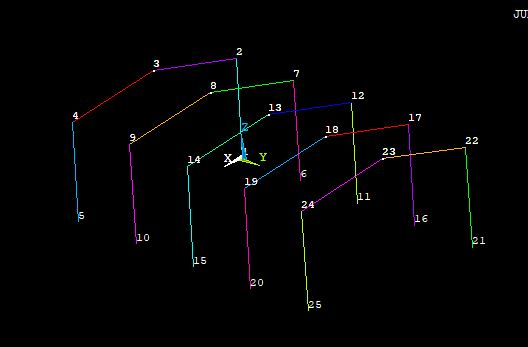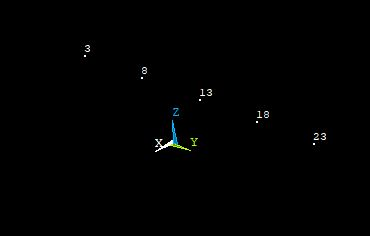## 3D Design

Topics related to Ansys Discovery and Ansys SpaceClaim.

•lilhaq93
Subscriber

Hi,

I'm a new user of Ansys so be clement.

I'm building a model by APDL commands.I want to connect few keypoint by lines.The thing is ,while I'm writing the input file I don't know the number of all my keypoints (some of them are created by KGEN or LGEN) but one thing I know for sure is one of their coordinates so I made a selection like this :KSEL,S,LOC,Z,6

KPLOTWhat I want to do now is to make a list(like array or table) of those keypoints (or of their coordinates) so that I can use a *DO to connect the first one to the second by LSTR ,and do the same for the rest of the keypoints.All this without knowing exactly the number of the keypoints

•jpasquerell
Ansys Employee

You could use the *VGET command 3 times to get the  x y and z values.

*dim,kplocs,array,n,3   ! replace n with max kp number

*vget,kplocs(1,1),kp,1,loc,x

*vget,kplocs(1,2),kp,1,loc,y

*vget,kplocs(1,3),kp,1,loc,z

but this will require a lot of manipulation to sort and get the needed info.  Are the lines at a regular interval?  If so make the first set of lines then use lgen to make the next set of keypoints and lines.  also, if the active csys is 0 then the lines are straight.   Another option in a do loop would be like this:

*do,jj,1,4,1                                   ! 4 loops with spacing of 1

lstr,kp(jj,ky(1),kz(1)), kp(jj,ky(2),kz(2))            ! kp(x,y,z) gets a keypoint number nearest the xyz coordinates and

! kx(k), ky(k) and kz(k) gets the coordinates of keypoint k

lstr,kp(jj,ky(2),kz(2)), kp(jj,ky(3),kz(3))

! repeat for other lines

*enddo

•jpasquerell
Ansys Employee

If your question is how to create lines going from 3 to 8, 8 to 13 then try:

*dim,klocs,array,5,2

km=0

*do,jj,1,5

km=kpnext(km)

klocs(jj,1)=km      ! minimum selected kp number

klocs(jj,2)=kx(km)   ! x coord of it

*enddo

*dim,oldo,array,5

*moper,oldo(1),klocs(1,1),sort,klocs(1,2)

*do,jj,1,4

lstr,klocs(jj),klocs(jj+1)

*enddo